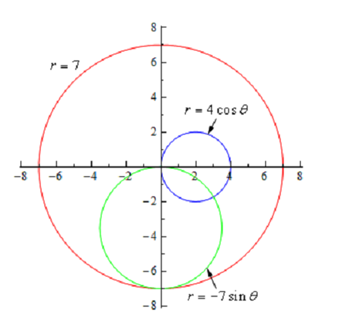## Example of circles - common polar coordinate graphs, Mathematics

Assignment Help:

Example of Circles - Common Polar Coordinate Graphs

Example: Graph r = 7, r = 4 cos θ, and r = -7 sin θ on similar axis system.

Solution

The very first one is a circle of radius 7 centered at the origin.  The 2nd is a circle of radius 2 centered at (2,0). The third is a circle of radius 7/2 centered at (0, - 7/2). Now here is the graph of three equations.#### Algebra, if a&b are aconsra

if a&b are aconsra

#### Simple interest, find the simple interest on Rs. 68,000 at 50/3 per annum f...

find the simple interest on Rs. 68,000 at 50/3 per annum for 9 month

#### #titconquent trianglr.., what is an isosceles triangle? ..

what is an isosceles triangle? ..

#### How would the society be strengthened, All things considered, in a sense of...

All things considered, in a sense of ethnicity (a sense of identification with and loyalty to one's group) good or bad? is it harmful or helpful? What would be lost if Americans lo

#### Calculate the number-average and weight-average molar mass, Three mixtures ...

Three mixtures were prepared with very narrow molar mass distribution polyisoprenesamples with molar masses of 8000, 25,000, and 100,000 as indicated below. (a) Equal numbers of

#### Round this number to the closest thousandth, It takes the moon an average o...

It takes the moon an average of 27.32167 days to circle the earth. Round this number to the closest thousandth. The thousandths place is the third digit to the right of the dec

#### Transportation problem, Define transportation problem

Define transportation problem

#### Statistics, How many 4 digit numbers can be formed using the numbers: 1 – 7...

How many 4 digit numbers can be formed using the numbers: 1 – 7. Repeated numbers CAN NOT be used

#### Project, report on shares and dovidend using newspaer

report on shares and dovidend using newspaer

#### Calculate the average, During 2008 the average number of beds required per ...

During 2008 the average number of beds required per day at St Hallam's hospital was 1800.  During the first 50 days of 2008 the average daily requirement for beds was 1830, with a